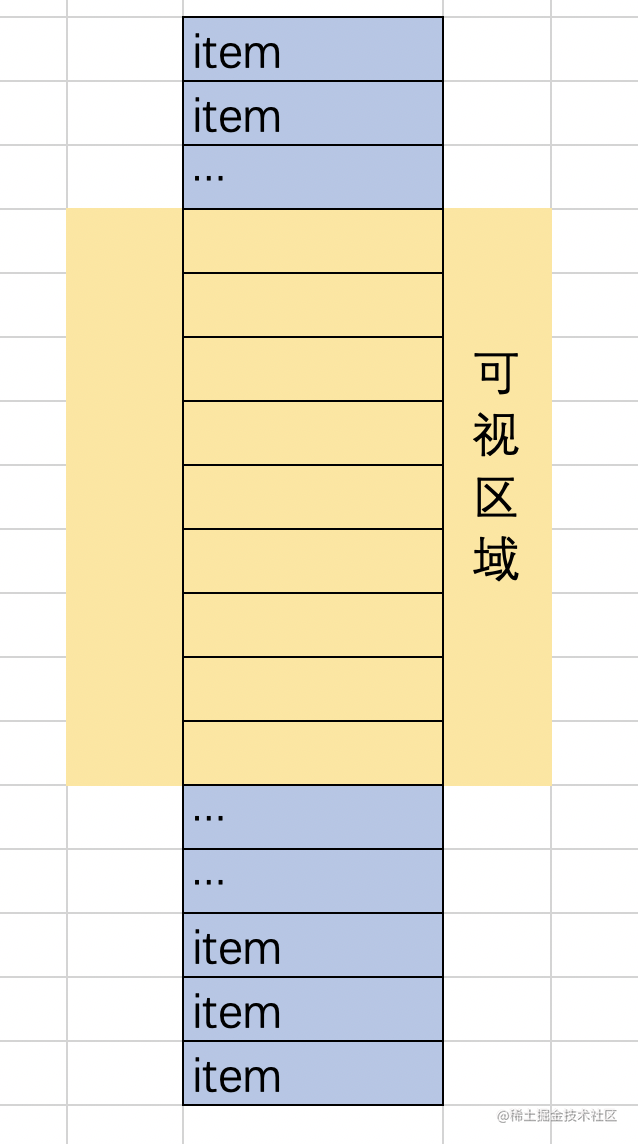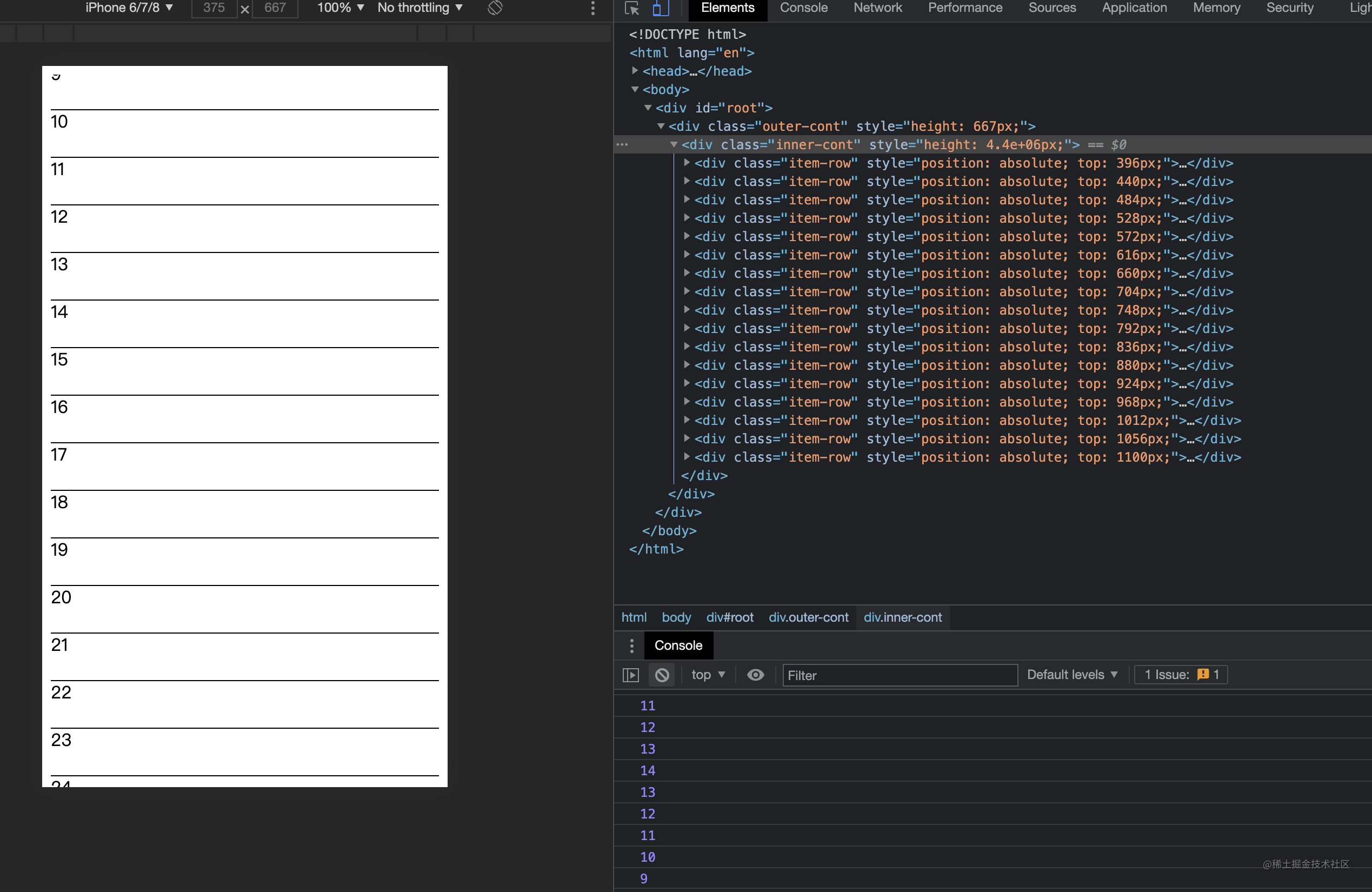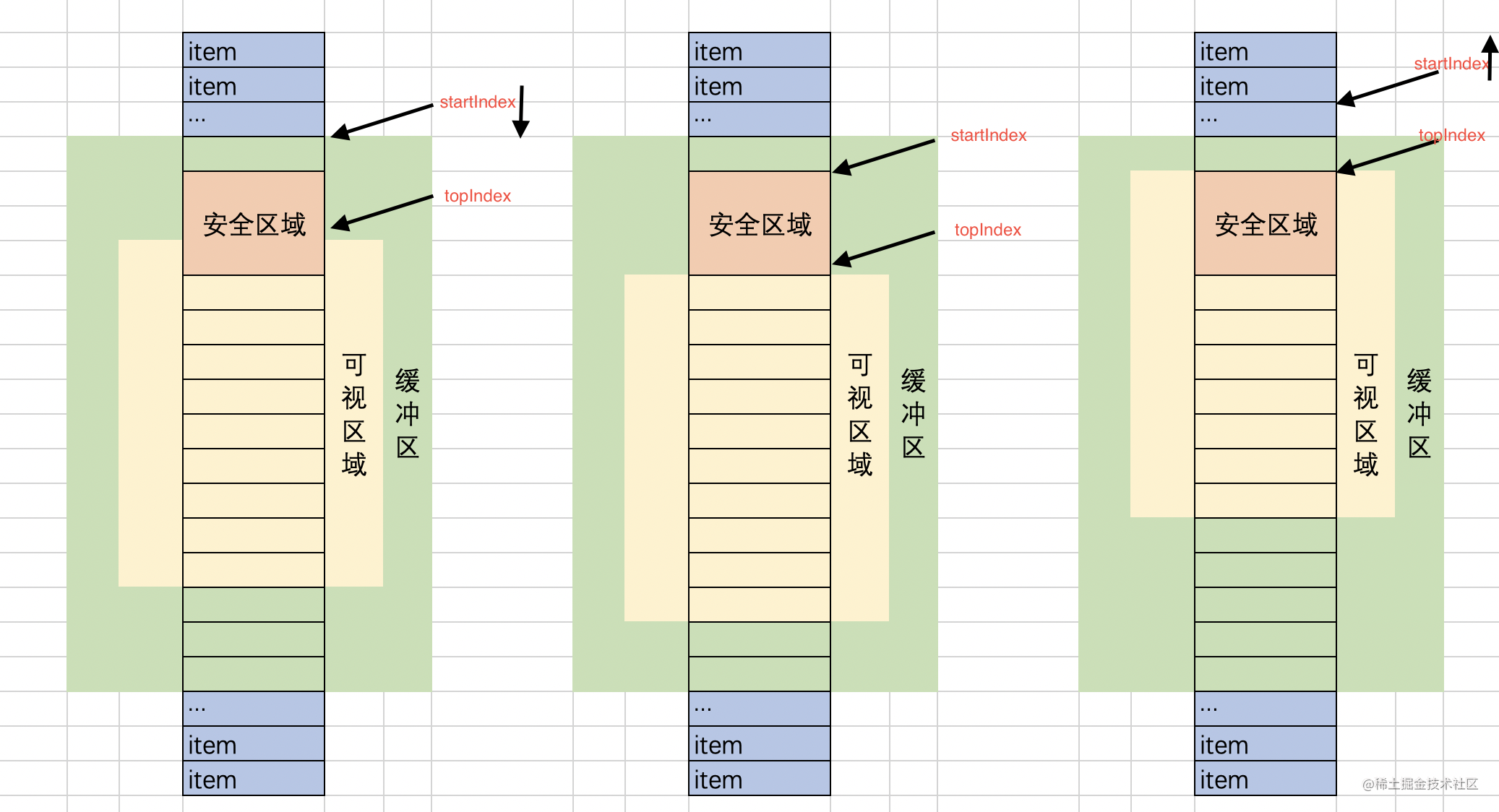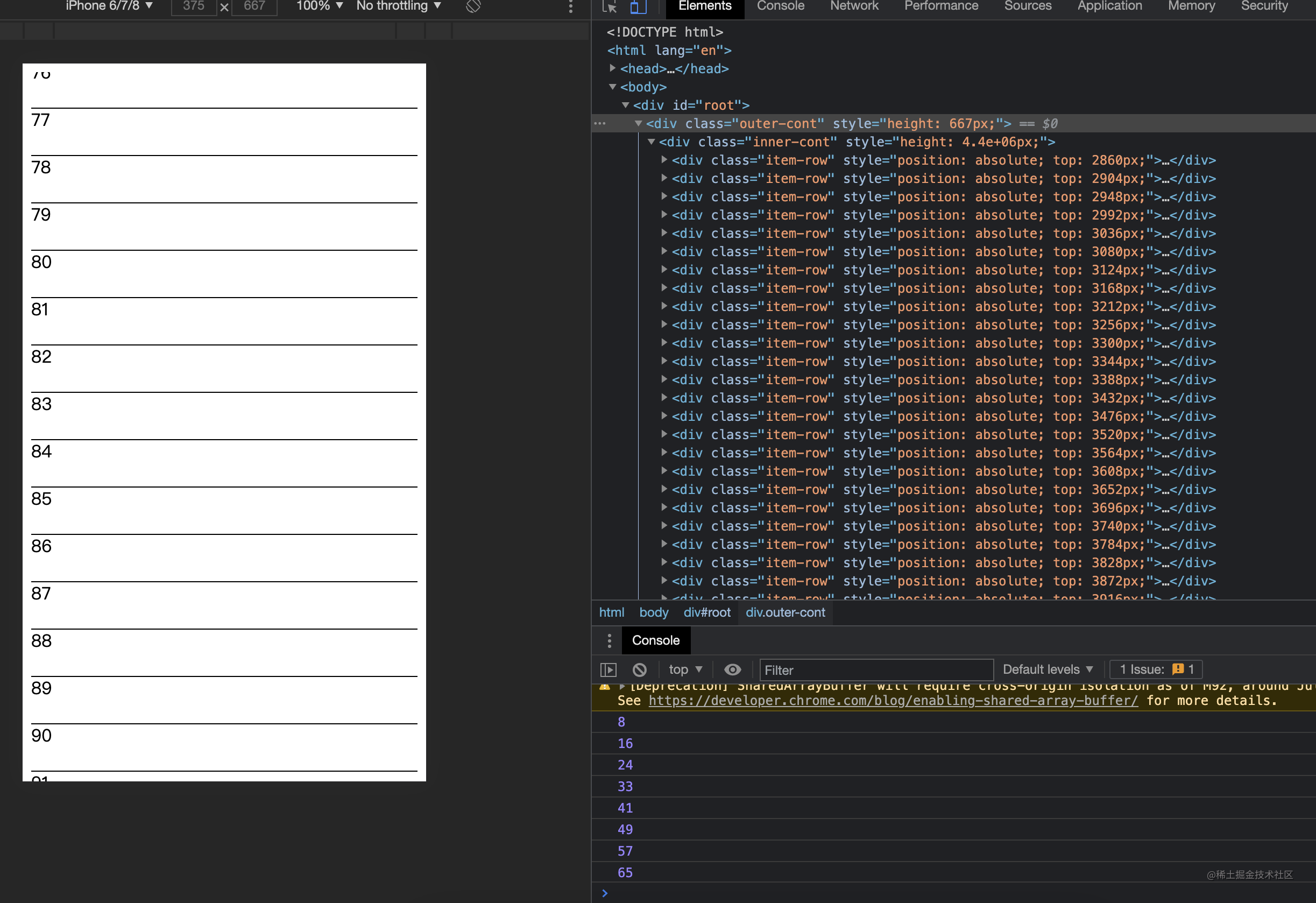二. 设计思路三. 代码设计

1.最简单实现

1. 开始数据的index，声明为startIndex
2. 结束数据的index，声明为endIndex

//./index.jsx
import React from 'react';
import './index.less';

let rowHeight = 44;

class TestList extends React.Component {
constructor(props) {
super(props);
let data = new Array(100000);
for (let index = 0; index < data.length; index++) {
data[index] = index;
}
this.allData = data;
this.state = {
dataSource: []
};
this.startIndex = 0;
}

componentDidMount() {
const containerH = document.documentElement.clientHeight;
this.size = Math.ceil(containerH / rowHeight) + 1;
this.endIndex = this.startIndex + this.size;
this.setState({
containerH,
dataSource: this.allData.slice(this.startIndex, this.endIndex)
})
}

scroll(e) {
//核心方法，下一步再写。
}

render() {
const { dataSource, containerH } = this.state;
const renderRow = (rowData, index) => {
const top = this.startIndex * rowHeight + rowHeight * index;
return (
<div key={rowData}
className='item-row'
style={{ position: 'absolute', top: top }}>
<span>{rowData}</span>
</div>
);
};
return (
<div
style={{ height: containerH }}
className='outer-cont'
onScroll={(e) => this.scroll(e)}
>
<div className="inner-cont" style={{ height: this.allData.length * rowHeight }}>
{dataSource.map((row, index) => renderRow(row, index))}
</div>
</div>
);
}
}

export default TestList;

//./index.less
.outer-cont {
position: relative;
overflow: auto;
.inner-cont {
position: relative;
.item-row {
width: 100%;
height: 44px;
border-bottom: 1px solid;
}
}
}

scroll(e) {
//如果数据量小于size，就不处理了
if (this.allData.length <= this.size) return;
const top = e.target.scrollTop;
//获取scrollTop，计算startIndex和endIndex
const topIndex = Math.floor(top / rowHeight);
//如果滑动量太小，index没有改变则不动。
if(this.startIndex === topIndex) return
this.startIndex = topIndex;
this.endIndex = this.startIndex + this.size;
//输出一下看看
console.log(this.startIndex);
//更新数据
this.setState({
dataSource: this.allData.slice(this.startIndex, this.endIndex)
})
}2. 缓冲区优化

1. 一开始的时候，可视区域还是在安全的区间内，在这个范围内移动，不需要刷新数据。
2. 当用户下滑，可视区域超出安全区域了，这个时候startIndex就需要向下移动。
1. 相反，startIndex就需要向上移动。
2. 其实2和3的情况不需要做区分，只需要在出了安全区域之后，移动startIndex，让topIndex落到安全区域即可，那就放在缓冲区域的最中间吧，这样算法就简单了， topIndex = startIndex + cacheSize/2。import React from "react";
import "./index.less";

let rowHeight = 44;

class TestList extends React.Component {
constructor(props) {
//和之前代码一样
...
this.cacheSize = 20;
}

componentDidMount() {
//初始化的时候加缓冲区
this.setState({
containerH,
dataSource: this.allData.slice(
this.startIndex,
this.endIndex + this.cacheSize
),
});
}

scroll(e) {
if (this.allData.length <= this.size) return;
const top = e.target.scrollTop;
let topIndex = Math.floor(top / rowHeight);
//判断是否出了安全区域，这块的数据可以自己调整
if (topIndex - this.startIndex < 3 || topIndex - this.startIndex > 17) {
//按照上面的分析，重置startIndex
let index = topIndex - cacheSize / 2 < 0 ? 0 : topIndex - this.cacheSize / 2;
if (this.startIndex === index) return;
this.startIndex = index;
console.log(this.startIndex);
this.endIndex = this.startIndex + this.size;
this.setState({
dataSource: this.allData.slice(
this.startIndex,
this.endIndex + this.cacheSize
),
});
}
}

render() {
//代码和之前一样
...
);
}
}

export default TestList;3.onScroll优化

debounce(fn, delay = 100) {
let timer = null;

return function (event) {
let context = this;
event.persist && event.persist();
if (timer) clearTimeout(timer);
timer = setTimeout(function () {
fn.call(context, event);
}, delay);
};
}